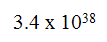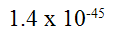# 8. Limits of floating point

Floating point is always contained within a fixed amount of bits. For example a 32 bit computer can conveniently use 4 bytes to represent a floating point number i.e. 32 bits

This means that there is a limit as to the range of numbers that can be represented.

Floating point was developed because it can cover a wide range and yet have reasonable precision. It can do so because the decimal point is allowed to 'float' compared to a fixed point scheme.

A 32 bit represenation is called 'single precision' and using the computer industry standard format for floating point (IEEE standard) the largest positive and negative number isOn the other hand the smallest positive and negative number that it can represent isThis is quite all right for most applications. But for scientific or engineering application running on the computer such as CAD or a simulation, there is the option to go to 'double precision' which is using 64 bits or 8 bytes. This vastly increases the range but then again it does run a lot slower.

`     `